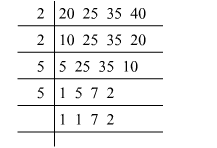# Find the least number which when divided by 20, 25, 35 and 40 leaves remaindersQuestion:

Find the least number which when divided by 20, 25, 35 and 40 leaves remainders 14, 19, 29 and 34 respectively.

Solution:

First find the LCM of 20, 25, 35 and 40.$\operatorname{LCM}(20,25,35,40)=2 \times 2 \times 2 \times 5 \times 5 \times 7=1400$

Now, we can see that

$20-14=6$

$25-19=6$

$35-29=6$

$40-34=6$

So, the required number would beLCM $(20,25,35,40)-6$

$=1400-6$

$=1394$